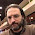## Wednesday, July 27, 2005

### Majority is Stablest

Consider the following two voting schemes to elect a single candidate.
1. Majority Vote.
2. A Majority of Majorities (think an electoral college system with states of equal size).
Which of these voting systems are more stable, i.e., less likely to be affected by flipping a small number of votes?

In an upcoming FOCS paper, Elchanan Mossel, Ryan O'Donnell and Krzysztof Oleszkiewicz prove the "Majority is Stablest" conjecture that answers the above question and in fact shows that majority is the most stable function among balanced Boolean functions where each input has low influence. To understand this result we'll need to define the terms in the statement of the theorem.

• Balanced: A Boolean function is balanced if it has the same number of inputs mapping to zero as mapping to one.
• The influence of the ith variable is the expectation over a random input of the variance of setting the ith bit of the input randomly. The conjecture requires the influence of each variable to be bounded by a small constant.
• Stability: The noise stability of f is the expectation of f(x)f(y) where x and y are chosen independently.
The majority is stablest conjecture has applications for approximation via the unique games conjecture.

1.I believe stability is defined with a parameter \epsilon
as E[f(x)f(y)] where for each i,
with probability 1-\epsilon y_i=x_i and otherwise y_i is chosen uniformly.

2.Also, it is an asymptotic theorem--the influences have to go to zero for it to say something interesting.

3.I keep seeing results about one-time voting systems, including instant-runoff voting or approval voting, but how about results where parties get to vote in multiple elections? (For example, real runoff elections.)

4.http://www.econ.boun.edu.tr/papers/pdf/wp-98-01.pdf

A Degree and an Efficiency of Manipulation of Known Social Choice Rules (Fuad Aleskerov, Eldeniz Kurbanov)

This paper compares some 24 voting methods.

fyi...

5.This result is related to some work in the study of voting power in political science; see here for some discussion and here for a discussion of various voting models and their interpretation. A key issue turns out to be modeling the correlations of votes for people who are near each other. (As far as I know, the results in pure math and cs assume independent voters.)